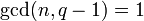Splitting criterion for conjugacy classes in special linear group of prime degree over a finite field

Statement

Suppose$n$ is a prime number and$q$ is a prime power (the underlying prime of$q$ may or may not be equal to$n$). Then, there is a finite field$\mathbb{F}_q$ of size$q$, unique up to isomorphism. We denote the special linear group$SL(n,\mathbb{F}_q)$ by$SL(n,q)$. Similarly, we denote the general linear group$GL(n,\mathbb{F}_q)$ by$GL(n,q)$.

Our goal is to determine the splitting criterion for elements between$GL(n,q)$ and the normal subgroup$SL(n,q)$. More explicitly, we are interested in the question: given an element$g \in SL(n,q)$, under what conditions is the$GL(n,q)$-conjugacy class of$g$ the same as its$SL(n,q)$-conjugacy class? Further, if they are not equal, how many different$SL(n,q)$-conjugacy classes does the$GL(n,q)$-conjugacy class split into?

Case of no nth roots of unity

If$\operatorname{gcd}(n,q - 1) = 1$ (this is the same as saying that$q$ is not 1 mod$n$), then none of the conjugacy classes split. In fact, in this case,$SL(n,q)$ is a direct factor of$GL(n,q)$, hence a conjugacy-closed subgroup; for more, see isomorphism between linear groups when degree power map is bijective.

Case of primitive nth roots of unity

If$\operatorname{gcd}(n,q - 1) = n$ (this is the same as saying that$q$ is 1 mod$n$), then the only conjugacy classes that split are the ones that comprise a single Jordan block of size$n$:

• There are$n$ such$GL(n,q)$-conjugacy classes in$SL(n,q)$. These correspond to eigenvalue choices that are the$n$ different$n^{th}$ roots of unity.
• Each such$GL(n,q)$-conjugacy class splits into$n$ conjugacy classes in$SL(n,q)$.
• Thus, there is a total of$n^2$ conjugacy classes in$SL(n,q)$ that we obtain after the splitting.

Particular cases

Value of prime$n$ Element structure of$SL(n,q)$ for general$q$
2 element structure of special linear group of degree two over a finite field
3 element structure of special linear group of degree three over a finite field